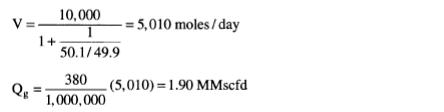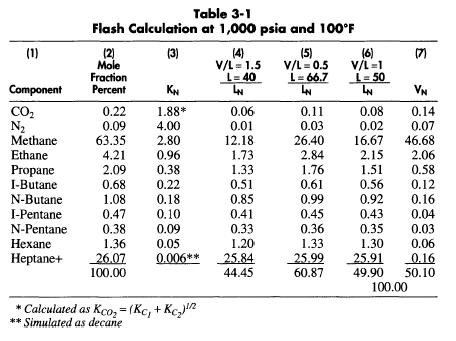# Gas Flow Rate

If the flow rate of the inlet stream is known in moles per day then the number of moles per day of gas flow can be determined from: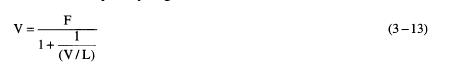where V = gas flow rate, moles/day
F = total stream flow rate, moles/day
L = liquid flow rate, moles/day
Derivation of Equation 3-13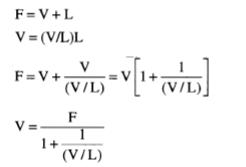Once the mole flow rate of gas is known, then the flow rate in standard cubic feet can be determined by recalling that one mole of gas occupies 380 cubic feet at standard conditions. Therefore: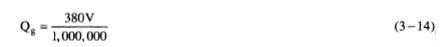where Qg = gas flow rate, MMscfd
Assuming a feed flow rate of 10,000 moles per day for the stream being flashed in Table 3-1: